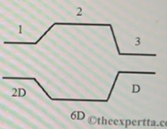# Problem: Water flows smoothly through a pipe with various circular cross-sections of diameters 2D, 6D, and D, respectively.Part (a) In which section is the volume flow rate largest? Choose the best answer.Part (b) In which section is the speed of the water largest? Choose the best answerPart (c) What is the ratio of the speed in section 3 to the speed in section 1?Part (d) In which section is the pressure largest? Choose the best answer

###### FREE Expert Solution

$\overline{){\mathbf{Av}}{\mathbf{=}}{\mathbf{c}}{\mathbf{o}}{\mathbf{n}}{\mathbf{s}}{\mathbf{t}}{\mathbf{a}}{\mathbf{n}}{\mathbf{t}}}$, where A is the cross-sectional area and v is the flow velocity.

Part (a)

The volumetric flow rate is constant throughout.

This is because the mass is constant.

84% (17 ratings)###### Problem Details

Water flows smoothly through a pipe with various circular cross-sections of diameters 2D, 6D, and D, respectively.Part (a) In which section is the volume flow rate largest? Choose the best answer.

Part (b) In which section is the speed of the water largest? Choose the best answer

Part (c) What is the ratio of the speed in section 3 to the speed in section 1?

Part (d) In which section is the pressure largest? Choose the best answer